﻿ 基于MATLAB的近似直线机构的解析分析与实现

# 基于MATLAB的近似直线机构的解析分析与实现Analysis and Implement of an Approximate Straight-Line Mechanism Based on MATLAB

Abstract: Because of less components, small cumulative error and high transmission efficiency, the ap-proximate straight-line mechanism plays an important role in metallurgy industrial and light in-dustry automatic production line, material vertical handling and transportation in logistics indus-try. Analytical method and mechanism theory applied to analyze the motion characteristics of the approximate straight-line mechanism. Differential size samples analyzed, implemented by MATLAB, it’s convenient for conceptual and scheme design of project.

1. 引言

2. 近似直线机构的运动轨迹分析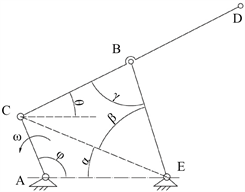α为铰点C和铰点E的连线CE与机架的夹角；β为连线CE与摇杆 $\stackrel{¯}{EB}$ 的夹角；γ为连杆 $\stackrel{¯}{CB}$ 与摇杆 $\stackrel{¯}{EB}$ 的夹角，此即为该机构的传动角；θ为连杆 $\stackrel{¯}{CB}$ (包含其延长线 $\stackrel{¯}{BD}$ )与水平线的夹角；φ为曲柄 $\stackrel{¯}{AC}$ 与机架 $\stackrel{¯}{AE}$ 的夹角(逆时针为正)； ${l}_{AC}$ 为曲柄 $\stackrel{¯}{AC}$ 的长度； ${l}_{CB}$ 为连杆 $\stackrel{¯}{CB}$ 的长度； ${l}_{EB}$ 为摇杆 $\stackrel{¯}{EB}$ 的长度； ${l}_{AE}$ 为机架 $\stackrel{¯}{AE}$ 的长度； ${l}_{BD}$ 为连杆 $\stackrel{¯}{CB}$ 延长线的长度。

Figure 1. Geometrical relationship of approximate straight-line mechanism

(1) 曲柄 $\stackrel{¯}{AC}$ 在0˚~180˚范围内

${l}_{CE}=\sqrt{{l}_{AC}^{2}+{l}_{AE}^{2}-2\cdot {l}_{AC}\cdot {l}_{AE}\mathrm{cos}\phi }$ (1)

$\alpha =\mathrm{arccos}\left(\frac{{l}_{CE}^{2}+{l}_{AE}^{2}-{l}_{AC}^{2}}{2\cdot {l}_{CE}\cdot {l}_{AE}}\right)$ (2)

$\beta =\mathrm{arccos}\left(\frac{{l}_{CE}^{2}+{l}_{EB}^{2}-{l}_{CB}^{2}}{2\cdot {l}_{CE}\cdot {l}_{EB}}\right)$ (3)

$\gamma =\mathrm{arccos}\left(\frac{{l}_{CB}^{2}+{l}_{EB}^{2}-{l}_{CE}^{2}}{2\cdot {l}_{CB}\cdot {l}_{EB}}\right)$ (4)

$\theta ={180}^{\circ }-\left(\alpha +\beta +\gamma \right)$ (5)

$\left\{\begin{array}{l}{x}_{D}={l}_{AC}\cdot \mathrm{cos}\phi +{l}_{CD}\cdot \mathrm{cos}\theta \\ {y}_{D}={l}_{AC}\cdot \mathrm{sin}\phi +{l}_{CD}\cdot \mathrm{sin}\theta \end{array}$ (6)

(2) 曲柄 $\stackrel{¯}{AC}$ 在180˚~360˚范围内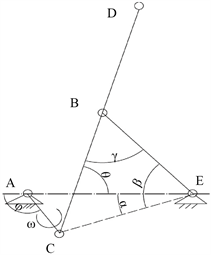Figure 2. Schematic geometrical relationship of approximate straight-line mechanism

$\theta ={180}^{\circ }-\left(\beta -\alpha +\gamma \right)$ (7)

$\left\{\begin{array}{l}{x}_{D}={l}_{AE}-{l}_{EB}\cdot \mathrm{cos}\left(\beta -\alpha \right)+{l}_{BD}\cdot \mathrm{cos}\theta \\ {y}_{D}={l}_{EB}\cdot \mathrm{sin}\left(\beta -\alpha \right)+{l}_{BD}\cdot \mathrm{sin}\theta \end{array}$ (8)

3. 摇杆 $\stackrel{¯}{EB}$ 的摆角分析

(1) φ在0˚~180˚时

$\psi ={180}^{\circ }-\left(\alpha +\beta \right)$ (9)

(2) φ在180˚~360˚时

$\psi ={180}^{\circ }-\left(\beta -\alpha \right)$ (10)

4. 案例分析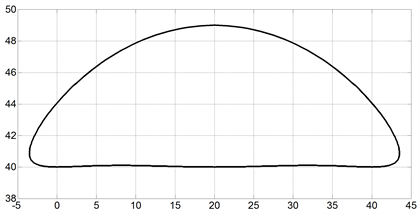Figure 3. Motion curve of point D ( $\phi \in \left[0˚,360˚\right]$ )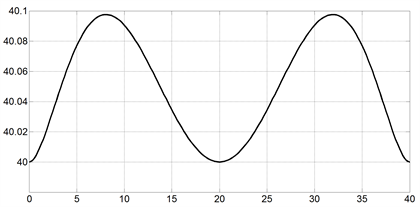Figure 4. Motion curve of point D ( $\phi \in \left[90˚,270˚\right]$ )Table 1. Results of analysis on two cases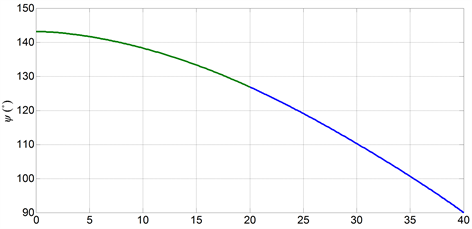Figure 5. Result of swing angle ψ of rocker $\stackrel{¯}{EB}$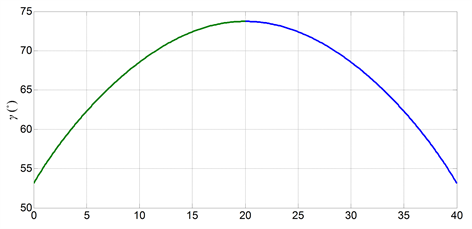Figure 6. Result of transmission angle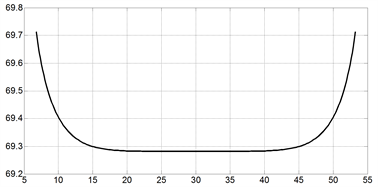Figure 7. Motion curve of point D ( $\phi \in \left[90˚,270˚\right]$ )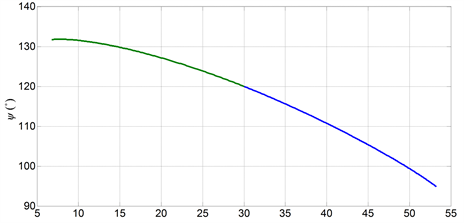Figure 8. Result of swing angle ψ of rocker $\stackrel{¯}{EB}$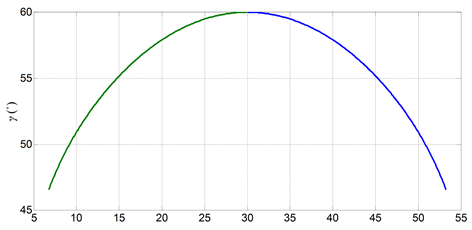Figure 9. Result of transmission angle

5. 结论

(1) 在同样的曲柄活动范围(即φ在90˚~270˚变化)，案例1的直线段长的区段比案例2的要大一些；案例1比案例2的要好。

(2) 摇杆 $\stackrel{¯}{EB}$ 的摆角输出，随尺寸的增大而输出相应的变小；案例1优于案例2。

(3) 传动角的变化趋势也是随尺寸增大而输出变小，这说明案例1的传动性能更佳。

(4) 案例1的综合性能比案例2更好，直线度更好一些，直线段的区域更大。

% MATLAB 实现程序

% AC为曲柄(主动件，φ为输入角)

% C、B、D在一条直线上；机架为AE；摇杆为EB

% CB=EB=BD=2.5*AC；AE=2*AC

%求D点的轨迹

clc;

AC=10;

CB=2.5.*AC;

EB=2.5.*AC;

BD=2.5.*AC;

AE=2.*AC;

phia=[0:2:180]*pi/180; %曲柄输入角在0到180°时

CE=sqrt(AC.*AC+AE.*AE-2.*AC.*AE*cos(phia)); %计算CE长度

alph=acos((CE.*CE+AE.*AE-AC.*AC)./(2.*CE.*AE)); %α

beta=acos((CE.*CE+EB.*EB-CB.*CB)./(2.*CE.*EB)); %β

gama =acos((CB.*CB+EB.*EB-CE.*CE)./(2.*CB.*EB)); %γ传动角

sita=pi-(alph+beta)-gama;

gama11= gama*180/pi;

baijiao=(pi-(alph+beta))*180/pi;%摇杆的摆角输出（°）

xd1=AC.*cos(phia)+(CB+BD).*cos(sita); %D点的横坐标

yd1=AC.*sin(phia)+(CB+BD).*sin(sita); %D点的纵坐标

phia1=[180:2:360]*pi/180; %当曲柄输入角在180°到360°时

CE1=sqrt(AC.*AC+AE.*AE-2.*AC.*AE*cos(2.*pi-phia1)); %计算CE长度

alph1=acos((CE1.*CE1+AE.*AE-AC.*AC)./(2.*CE1.*AE)); %α

beta1=acos((CE1.*CE1+EB.*EB-CB.*CB)./(2.*CE1.*EB)); %β

gama1=acos((CB.*CB+EB.*EB-CE1.*CE1)./(2.*CB.*EB)); %γ传动角

sita1=pi-(beta1-alph1)-gama1; %注意：几何关系变化

gama11= gama1*180/pi;

baijiao1=(pi-( beta1-alph1))*180/pi; %摇杆的摆角输出（°）

xd2=AE-EB.*cos(beta1-alph1)+BD.*cos(sita1); %D点的横坐标

yd2=EB.*sin(beta1-alph1)+BD.*sin(sita1); %D点的纵坐标

plot(xd1,yd1,xd2,yd2)%绘制轨迹图

grid

 倪庆兴, 王焕勇. 起重机械[M]. 上海: 上海交通大学出版社, 1990.

 李克勤. 具有近似水平直线运动轨迹双摇杆变幅机构的运动学分析与计算[J]. 港口装卸, 2002(2): 5-7.

 陈国华. 机械机构及应用[M]. 第2版. 北京: 机械工业出版社, 2013.

 杨黎明, 杨志勤. 机构选型与运动设计[M]. 北京: 国防工业出版社, 2007.

 Ullman, D.G. (2010) The Mechanical Process. 4th Edition, The McGraw-Hill Companies, Inc., New York.

 黄锡恺, 郑文纬. 机械原理[M]. 第6版. 北京: 高等教育出版社, 1989.

 曹惟庆, 徐曾荫. 机构设计[M]. 第2版. 北京: 机械工业出版社, 1999.

 杨基厚. 机构运动学与动力学[M]. 北京: 机械工业出版社, 1987.

 李琦, 李华英. 平面连杆机构连杆曲线的直线近似度研究[J]. 轻工机械, 2003(2): 39-41.

 王忠, 朱洪俊, 廖磊, 等. 瞬心无穷远时近似直线轨迹的四杆机构综合[J]. 机械工程学报, 2007(10): 46-49.

 钱卫香, 韩建友. 特殊位形下近似直线轨迹连杆机构综合[J]. 机械设计与研究, 2007(3): 40-43.

 Dijksman, E.A. and Smals, A.T.J.M. (1996) λ-Formed 4-bar Linkages Set in a Translation-Position to Design Mechanisms Approximating a Straight Line. Mechanism and Machine Theory, 31, 1033-1042.
https://doi.org/10.1016/0094-114X(96)84596-4

Top